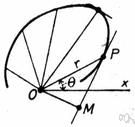# polar coordinate

Also found in: Thesaurus, Wikipedia.
Related to polar coordinate: spherical coordinate

## polar coordinate

n.
Either of two coordinates, the radius or the polar angle, that together specify the position of a point in a plane.
ThesaurusAntonymsRelated WordsSynonymsLegend:
 Noun 1polar coordinate - either of two values that locate a point on a plane by its distance from a fixed pole and its angle from a fixed line passing through the polecoordinate, co-ordinate - a number that identifies a position relative to an axis
Based on WordNet 3.0, Farlex clipart collection. © 2003-2012 Princeton University, Farlex Inc.
References in periodicals archive ?
Goal Scoring in Soccer: A Polar Coordinate Analysis of Motor Skills Used by Lionel Messi.
(2009), or a polar coordinate system as in Wang and Rose (2005), the governing equation is recast in terms of a local curvilinear system, formed by the curvilinear coordinate s along the wall and the local wall radius of curvature [r.sub.cw].
Alternatively, a polar coordinate system can be used for targeting, with the needle angled slightly upwards as opposed to perpendicular in the Cartesian coordinate system.
In this paper, PSSE is considered in the rectangular and polar coordinate systems.
We choose the dependence [R.sub.r]([phi]) of the oval-shaped rim radius with the eccentricity e on the polar coordinate [phi] as
These transform functions are Cartesian coordinate transformation, polar coordinate transformation and function transformation.
where [mathematical expression not reproducible] represents the angular polar coordinate corresponding to particular pixel (i, j) [member of] M and R(i ,j), RE) represents the distance between the pixel (i, j) [epsilon] and the RE:
, as the original data is in the polar coordinate system, an interpolation method was utilized to transform the polar coordinate system into the three-dimensional Cartesian coordinate system.
Prior to this, we consider a generalized polar coordinate transformation allowing one of the specific representations derived later on.
As based on the analysis of the key reasons for the inefficiency of search and poor convergence, this research proposes a novel approach that transforms the coordinate system for design variables and constraint space to local polar coordinate systems.
A crucial step here is transformation of the amplitude spectra into the polar coordinate system to obtain functions [A.sup.p.sub.1]; [A.sup.p.sub.2] : [R.sup.+.sub.0] x (0; 2[pi]) [right arrow] [R.sup.+.sub.-] such that [A.sup.p.sub.1]([rho];[phi]) = [A.sup.p.sub.2]([rho]; ([phi] + [theta]).
Bear  proved that if r = [K.sub.q.sup.1/2] or r = [K.sub.j.sup.-1/2] in the polar coordinate system, mapping can form an ellipse (under the condition of three-dimensional ellipsoid), where r represents radius vector, [K.sub.q] represents permeability coefficient in the direction of the flow line, and [K.sub.j] represents permeability coefficient in the direction of the hydraulic gradient.

Site: Follow: Share:
Open / Close